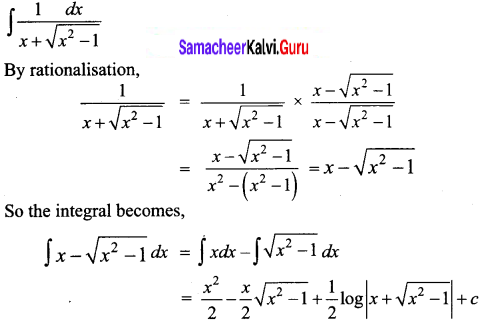Students can download 12th Business Maths Chapter 2 Integral Calculus I Ex 2.7 Questions and Answers, Samacheer Kalvi 12th Business Maths Book Solutions Guide Pdf helps you to revise the complete Tamilnadu State Board New Syllabus and score more marks in your examinations.

## Tamilnadu Samacheer Kalvi 12th Business Maths Solutions Chapter 2 Integral Calculus I Ex 2.7

Integrate the following with respect to x.

Question 1.
$$\frac{1}{9-16 x^{2}}$$
Solution: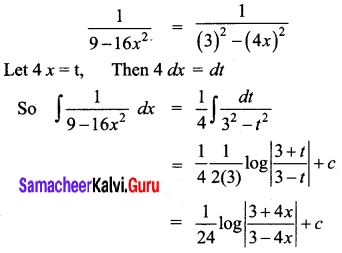Question 2.
$$\frac{1}{9-8 x-x^{2}}$$
Solution: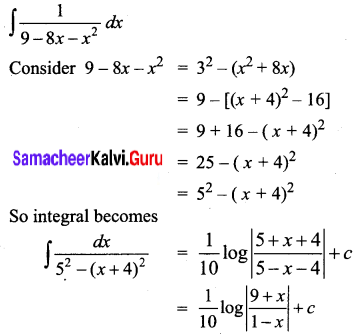Question 3.
$$\frac{1}{2 x^{2}-9}$$
Solution: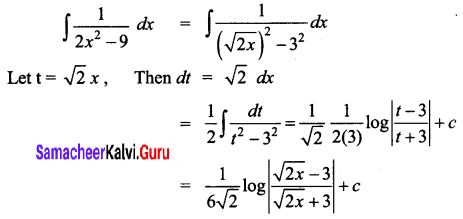Question 4.
$$\frac{1}{x^{2}-x-2}$$
Solution: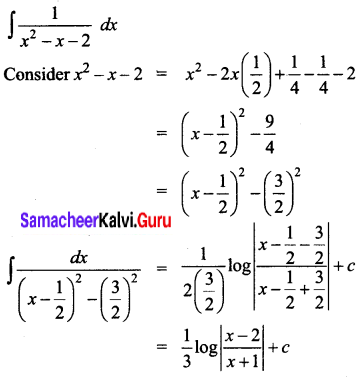Question 5.
$$\frac{1}{x^{2}+3 x+2}$$
Solution: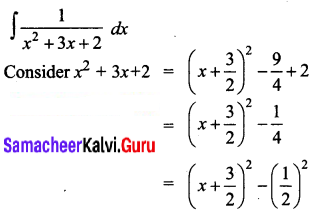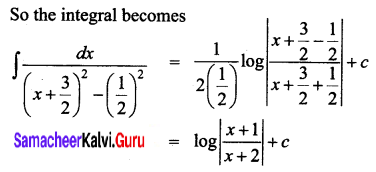Question 6.
$$\frac{1}{2 x^{2}+6 x-8}$$
Solution: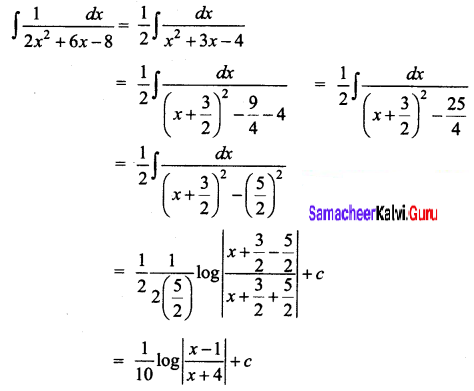Question 7.
$$\frac{e^{x}}{e^{2 x}-9}$$
Solution: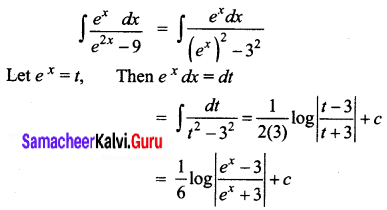Question 8.
$$\frac{1}{\sqrt{9 x^{2}-7}}$$
Solution: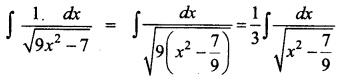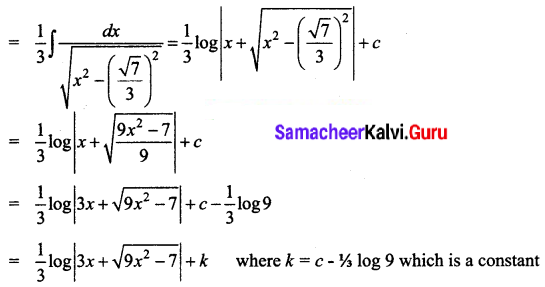Question 9.
$$\left(\frac{1}{\sqrt{x^{2}+6 x+13}}\right)$$
Solution: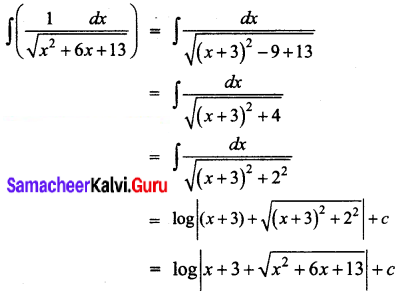Question 10.
$$\left(\frac{1}{\sqrt{x^{2}-3 x+2}}\right)$$
Solution: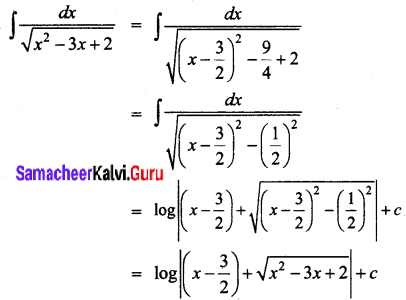Question 11.
$$\frac{x^{3}}{\sqrt{x^{8}-1}}$$
Solution: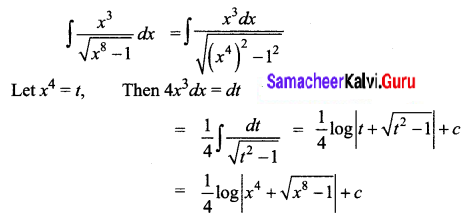Question 12.
$$\sqrt{1+x+x^{2}}$$
Solution: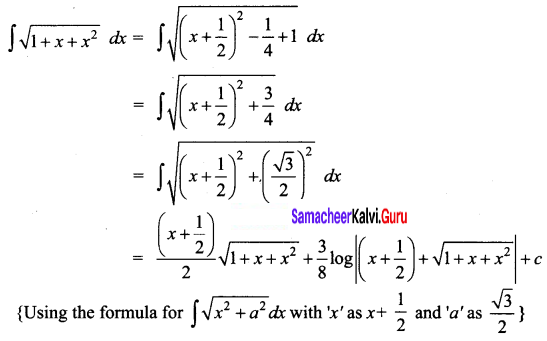Question 13.
$$\sqrt{x^{2}-2}$$
Solution: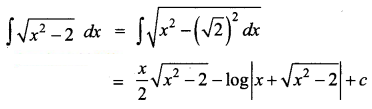Question 14.
$$\sqrt{4 x^{2}-5}$$
Solution: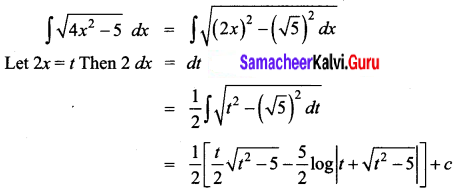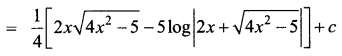Question 15.
$$\sqrt{2 x^{2}+4 x+1}$$
Solution: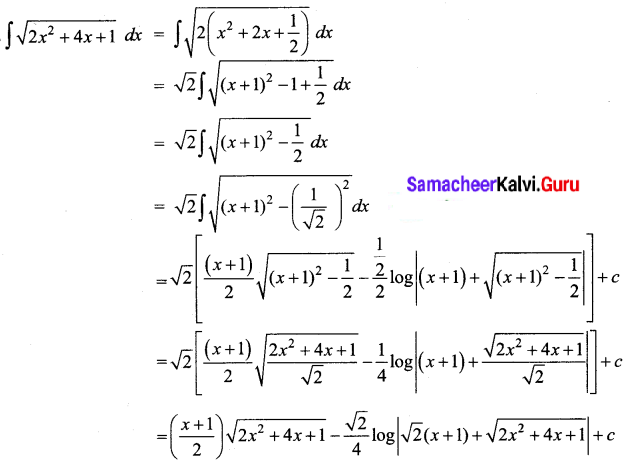Question 16.
$$\frac{1}{x+\sqrt{x^{2}-1}}$$
Solution: